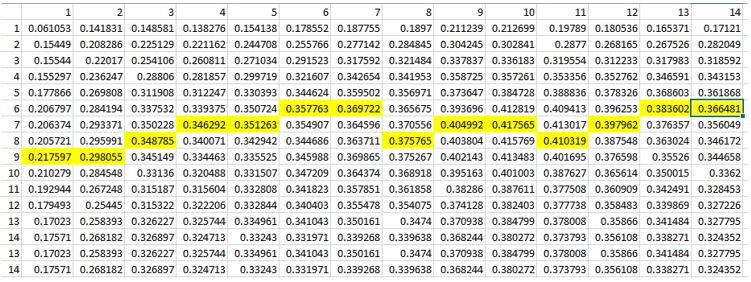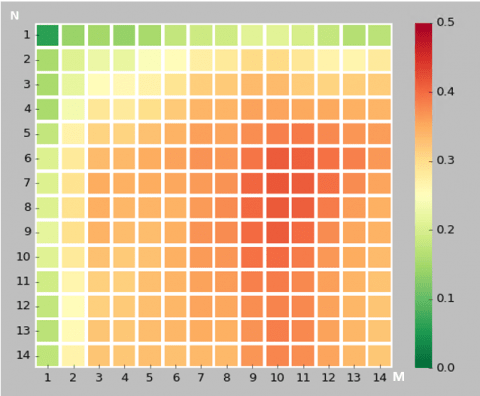## Fibonacci Stock Trading – Using Fibonacci Retracement for Stock Market Prediction

As stated on allstarcharts.com by expert with more than 10 years, Fibonacci Analysis is one of the most valuable and easy to use tools for stock market technical analysis. And Fibonacci tools can be applied to longer-term as well as to short-term. 

In this post we will take a look how Fibonacci numbers can help to stock market analysis. For this we will use different daily stock prices data charts with added Fibonacci lines.Just for the references – The Fibonacci numbers (or Fibonacci sequence), are numbers that after the first two are the sum of the two preceding ones : 0, 1, 1, 2, 3, 5, 8, 13, 21, 34, 55 …

According to “Magic of Fibonacci Sequence in Prediction of Stock Behavior”  Fibonacci series are widely used in financial market to predict the resistance and support levels through Fibonacci retracement. In this method major peak and trough are identified, then it is followed by dividing the vertical distance into 23.6%, 38.2%, 50%, 61.8% and 100%. These percentage numbers (except 50%) are obtained by dividing element in Fibonacci sequence by its successors for example 13/55=0.236. 
Ratio of two successive numbers of Fibonacci sequence is approximately 1.618034, so if we multiply 23.6 by 1.618034 we will get next level number 38.2.

## 5 Fibonacci Chart Examples

Now let’s look at charts with added Fibonacci lines (23.6%, 38.2%, 50%, 61.8% and 100%).

Below are 5 daily the stock market price charts for different stock tickers. Fibonacci line numbers are shown on the right side, and stock ticker is on the top of chart.

Here we can see Fibonacci line at 61.8 is a support line

This chart is for the same symbol but for smaller time frame. 23.6 line is support and then resistance line

Here are Fibonacci Retracement lines at 23.6 and 61.8 line up well with support and resistance areas

Fibonacci Retracement 61.8 is support line

Fibonacci Retracement lines such as 23.6, 38.2, 61.8 line up well with support and resistance areas

## Conclusion

After reviewing above charts we can say that Fibonacci Retracement can indicate potential support and resistance levels. The trend often is changing in such areas. So Fibonacci retracement can be used for stock market prediction.

Do you use Fibonacci tools? Feel free to put in the comments how do you apply Fibonacci retracements in stock trading. Do you find Fibonacci tools helpful or not? As always any feedback is welcome.

## Machine Learning for Correlation Data Analysis Between Food and Mood

Can sweet food affect our mood? A friend of mine was interesting if some of his minor mood changes are caused by sugar intake from sweets like cookies. He collected and provided records and in this post we will use correlation data analysis with python pandas dataframes to check the connection between food and mood. We will create python script for this task.## Connection Between Eating and Mental Health

From internet resources we can confirm that relationship between how we feel and what we eat exists. Sweet food is not recommended to eat as fluctuations in blood sugar cause mood swings, lack of energy . The information about chocolate is however contradictory. Chocolate affects us both negatively and positively. But chocolate has also sugar.
What if we eat only small amount of sweets and not each day – is there still any connection and how strong is it? The machine learning data analysis can help us to investigate this.

## The Problem

So in this post we will estimate correlation between sweet food and mood based on provided daily data.
Correlation means association – more precisely it is a measure of the extent to which two variables are related. 

## Data

The dataset has two columns, X and Y where:
X is how much sweet food was taken on daily basis, on the scale 0 – 1 , 0 is nothing, 1 means a max value.
Y is variation of mood from optimal state, on the scale 0 – 1 , 0 – means no variations or no defects, 1 means a max value.

## Approach

If we calculate correlation between 2 columns of daily data we will get something around 0. However this would not show whole picture. Because the effect of the food might take action in a few days. The good or bad feeling can also stay for few days after the event that caused this feeling.
So we would need to take average data for several days for both X (looking back) and Y (looking forward). Here is the diagram that explains how data will be aggregated:

And here is how we can do this in the program:
1.for each day take average X data for last N days and take average Y data for M next days.
2.create a pandas dataframe which has now new moving averages for X and Y.
3.calculate correlation between new X and Y data

What should be N and M? We will use different values – from 1 to 14. And we will check what is the highest value for correlation.

Here is the python code to use pandas dataframe for calculating averages:

```def get_data (df_pandas,k,z):

x = np.zeros(df_pandas.shape)
y = np.zeros(df_pandas.shape)

new_df = pd.DataFrame() #creates a new dataframe that's empty
for index, row in df_pandas.iterrows():

x[index]=df_pandas.loc[index-k:index,'X'].mean()

y[index]=df_pandas.loc[index:index+z,'Y'].mean()

new_df=pd.concat([pd.DataFrame(x),pd.DataFrame(y)], "columns")
new_df.columns = ['X', 'Y']

return new_df

```

## Correlation Data Analysis

For calculating correlation we use also pandas dataframe. Here is the code snipped for this:

```for i in range (1,n):
for j in range (1,m):

data=get_data(df, i, j)
corr_df.loc[i, j] = data['X'].corr(data['Y'])

print ("corr_df")
print (corr_df)
```

pandas.DataFrame.corr by default is calculating pearson correlation coefficient – it is the measure of the strength of the linear relationship between two variables. In our code we use this default option. 

## Results

After calculating correlation coefficients we output data in the table format and plot results on heatmap using seaborn module. Below is the data output and the plot. The max value of correlation for each column is highlighted in yellow in the data table. Input data and full source code are available at ,.Correlation data between sweet food (taken in n days) and mood (in next m days)Correlation data between sweet food (taken in N days) and mood in the following M days, averaged

## Conclusion

We performed correlation analysis between eating sweet food and mental health. And we confirmed that in our data example there is a moderate correlation (0.4). This correlation is showing up when we use moving averaging for 5 or 6 days. This corresponds with observation that swing mood may appear in several days, not on the same or next day after eating sweet food.

We also learned how we can estimate correlation between two time series variables X, Y.